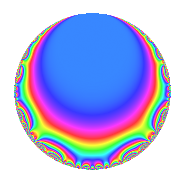# Properties

 Label 111.1.d.bLevel 111 Weight 1 Character orbit 111.d Self dual Yes Analytic conductor 0.055 Analytic rank 0 Dimension 2 Projective image $$D_{4}$$ CM disc. -111 Inner twists 4

# Related objects

## Newspace parameters

 Level: $$N$$ = $$111 = 3 \cdot 37$$ Weight: $$k$$ = $$1$$ Character orbit: $$[\chi]$$ = 111.d (of order $$2$$ and degree $$1$$)

## Newform invariants

 Self dual: Yes Analytic conductor: $$0.0553962164023$$ Analytic rank: $$0$$ Dimension: $$2$$ Coefficient field: $$\Q(\sqrt{2})$$ Coefficient ring: $$\Z[a_1, a_2]$$ Coefficient ring index: $$1$$ Projective image $$D_{4}$$ Projective field Galois closure of 4.0.333.1 Artin image size $$16$$ Artin image $D_8$ Artin field Galois closure of 8.0.4102893.1

## $q$-expansion

Coefficients of the $$q$$-expansion are expressed in terms of $$\beta = \sqrt{2}$$. We also show the integral $$q$$-expansion of the trace form.

 $$f(q)$$ $$=$$ $$q -\beta q^{2} - q^{3} + q^{4} + \beta q^{5} + \beta q^{6} + q^{9} +O(q^{10})$$ $$q -\beta q^{2} - q^{3} + q^{4} + \beta q^{5} + \beta q^{6} + q^{9} -2 q^{10} - q^{12} -\beta q^{15} - q^{16} -\beta q^{17} -\beta q^{18} + \beta q^{20} + \beta q^{23} + q^{25} - q^{27} -\beta q^{29} + 2 q^{30} + \beta q^{32} + 2 q^{34} + q^{36} - q^{37} + \beta q^{45} -2 q^{46} + q^{48} - q^{49} -\beta q^{50} + \beta q^{51} + \beta q^{54} + 2 q^{58} -\beta q^{59} -\beta q^{60} - q^{64} -\beta q^{68} -\beta q^{69} + \beta q^{74} - q^{75} -\beta q^{80} + q^{81} -2 q^{85} + \beta q^{87} + \beta q^{89} -2 q^{90} + \beta q^{92} -\beta q^{96} + \beta q^{98} +O(q^{100})$$ $$\operatorname{Tr}(f)(q)$$ $$=$$ $$2q - 2q^{3} + 2q^{4} + 2q^{9} + O(q^{10})$$ $$2q - 2q^{3} + 2q^{4} + 2q^{9} - 4q^{10} - 2q^{12} - 2q^{16} + 2q^{25} - 2q^{27} + 4q^{30} + 4q^{34} + 2q^{36} - 2q^{37} - 4q^{46} + 2q^{48} - 2q^{49} + 4q^{58} - 2q^{64} - 2q^{75} + 2q^{81} - 4q^{85} - 4q^{90} + O(q^{100})$$

## Character Values

We give the values of $$\chi$$ on generators for $$\left(\mathbb{Z}/111\mathbb{Z}\right)^\times$$.

 $$n$$ $$38$$ $$76$$ $$\chi(n)$$ $$-1$$ $$-1$$

## Embeddings

For each embedding $$\iota_m$$ of the coefficient field, the values $$\iota_m(a_n)$$ are shown below.

For more information on an embedded modular form you can click on its label.

Label $$\iota_m(\nu)$$ $$a_{2}$$ $$a_{3}$$ $$a_{4}$$ $$a_{5}$$ $$a_{6}$$ $$a_{7}$$ $$a_{8}$$ $$a_{9}$$ $$a_{10}$$
110.1
 1.41421 −1.41421
−1.41421 −1.00000 1.00000 1.41421 1.41421 0 0 1.00000 −2.00000
110.2 1.41421 −1.00000 1.00000 −1.41421 −1.41421 0 0 1.00000 −2.00000
 $$n$$: e.g. 2-40 or 990-1000 Significant digits: Format: Complex embeddings Normalized embeddings Satake parameters Satake angles

## Inner twists

Char. orbit Parity Mult. Self Twist Proved
1.a Even 1 trivial yes
111.d Odd 1 CM by $$\Q(\sqrt{-111})$$ yes
3.b Odd 1 yes
37.b Even 1 yes

## Hecke kernels

This newform can be constructed as the kernel of the linear operator $$T_{2}^{2} - 2$$ acting on $$S_{1}^{\mathrm{new}}(111, [\chi])$$.